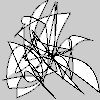# Py5Shape.bezier_vertices()#

Create a collection of bezier vertices.

## Examples#import numpy as np

def setup():
py5.size(100, 100, py5.P2D)
random_bezier_vertices = 100 * np.random.rand(25, 6)
s = py5.create_shape()
with s.begin_shape():
s.vertex(py5.width / 2, py5.height / 2)
s.bezier_vertices(random_bezier_vertices)
py5.shape(s)


## Description#

Create a collection of bezier vertices. The purpose of this method is to provide an alternative to repeatedly calling Py5Shape.bezier_vertex() in a loop. For a large number of bezier vertices, the performance of bezier_vertices() will be much faster.

The coordinates parameter should be a numpy array with one row for each bezier vertex. The first few columns are for the first control point, the next few columns are for the second control point, and the final few columns are for the anchor point. There should be six or nine columns for 2D or 3D points, respectively.

Drawing 2D bezier curves requires using the P2D renderer and drawing 3D bezier curves requires using the P3D renderer. When drawing directly with Py5Shape objects, bezier curves do not work at all using the default renderer.

This method can only be used within a Py5Shape.begin_shape() and Py5Shape.end_shape() pair.

## Signatures#

bezier_vertices(
coordinates: npt.NDArray[np.floating],  # 2D array of bezier vertex coordinates with 6 or 9 columns for 2D or 3D points, respectively
/,
) -> None


Updated on June 26, 2023 01:48:37am UTC